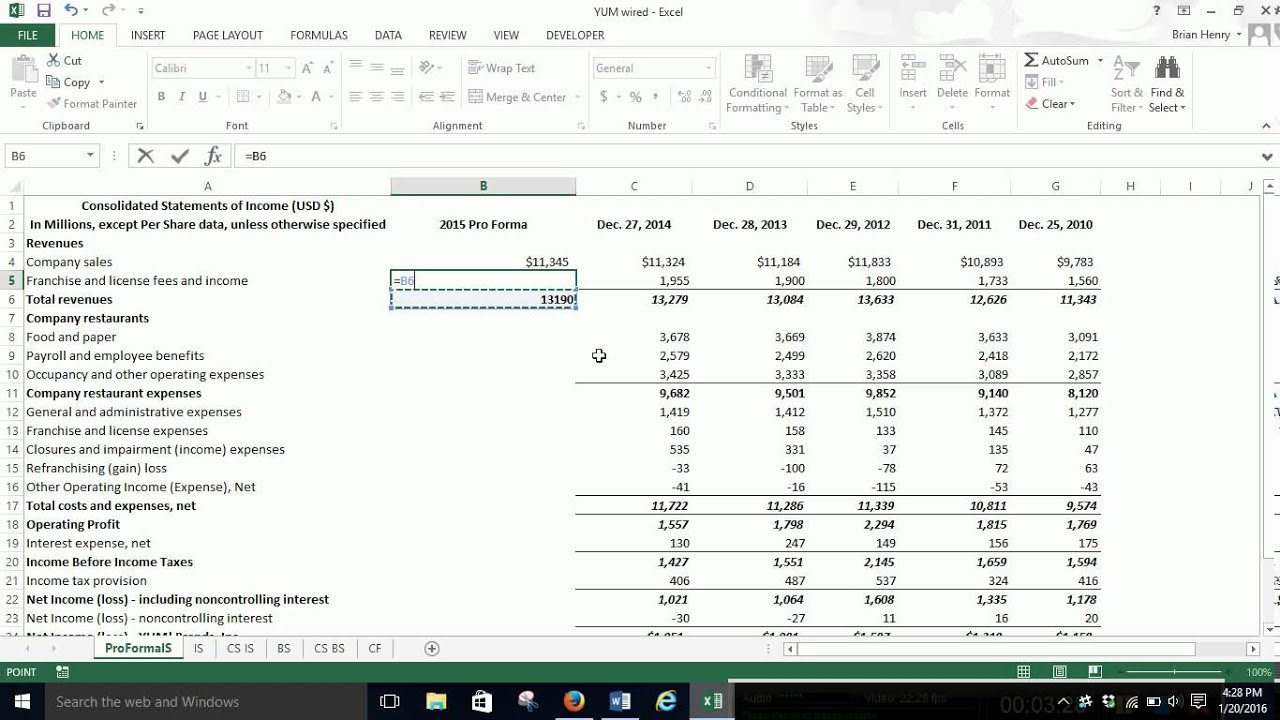# Financial Forecasting Using Percent of Sales

Feb 25, 2020 • edited Feb 26, 2020Financial forecasting often begins with a forecast of future sales. The Sales Forecast serves as the basis for estimating future expenses, assets, and liabilities. Many of these accounts vary with changes in sales. Therefore, using a percent (%) of sales can be very useful for forecasting a Balance Sheet.

The following steps can be used to prepare a forecasted (pro-forma) Balance Sheet based on the % of Sales Method:

1. Determine which Balance Sheet accounts vary with Sales (such as accounts receivable). Calculate the % of sales for each account that varies with sales.

2. For accounts that do not vary with sales (such as long-term debt and equity), simply list the current balances from the last Balance Sheet.

3. Calculate the future Retained Earnings balance by adding projected net income and subtracting any future dividends from the Beginning Balance for Retained Earnings. Don't forget to calculate a % of sales for Net Income and Dividends.

4. Add up your assets to determine total projected assets. Now add up your liabilities and equity to determine the financing of assets. If total assets are greater than total liabilities and equity, then you will need to raise additional capital.

#Tutorial#Financial Forecasting

How to use the AVERAGE function in Excel

How to use Today's Date With Worksheet in Excel